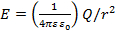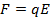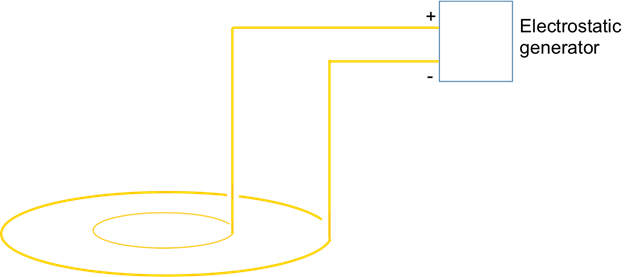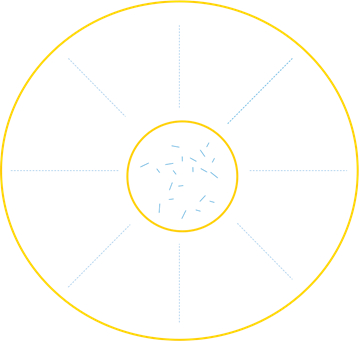Trial ends in

# Electric Fields

### Overview

Source: Yong P. Chen, PhD, Department of Physics & Astronomy, College of Science, Purdue University, West Lafayette, IN

An electric field is generated by a charged object (referred to as the source charge) in the space around it, and represents the ability to exert electric force on another charged object (referred to as the test charge). Represented by a vector at any given point in the space, the electric field is the electrical force per unit test charge placed at that point (the force on an arbitrary charge would be the charge times the electric field). The electric field is fundamental to electricity and effects of charges, and it is also closely related to other important quantities such as electrical voltage.

This experiment will use electrified powders in an oil that line up with electric fields produced by charged electrodes to visualize the electric field lines. This experiment will also demonstrate how an electric field can induce charges and how charges respond to the electric field by observing the effect of a charged rod on a nearby soda can.

### Principles

A charged object produces an electric field in the surrounding space. For example, according to the Gauss's law, a point charge Q located at the origin produces an electric field:(Equation 1)

at any point in the space with a distance r from the charge (at origin r = 0), and the direction of the electric field is along the radial direction (away from the charge if Q is positive, and towards the charge if Q is negative). A collection of charges would produce a total electric field according to the superposition principle, namely the total electric field is the vector sum of the electric fields produced by individual charges. For a uniformly charged sphere with total charge Q, the electric field produced outside the sphere is the same as the electric field (given by Equation 1) due to a point-like charge Q located at the center of the sphere, whereas the electric field inside the sphere would be zero.

If one follows the local direction of the electric field to trace out the vector field lines, these lines (whose tangent reflects the local direction of the electric field, and the density of the lines reflects the strength of the local electric field) are known as "electric field lines". They are fictitious lines that help visualize the distribution and direction of electric fields.

An electric field is closely related to electric potential. An electric field would produce a potential drop (or "voltage drop") along the direction of the field. Conversely, a convenient way to generate an electric field is to apply a potential difference. For example, if two different voltages are applied on two separated conductors (or a nonzero voltage applied on a conductor, while keeping another conductor "grounded" at zero voltage), then an electric field in the space between the two conductors pointing in the direction from the higher voltage conductor to the lower voltage conductor is generated.

An electric field (E) will exert a force,on a charge (q). The direction of the force is the same as the electric field for positive q, and opposite to the electric field for negative q. If a conductor (such as a metal) containing mobile charges is placed in an electric field, the electric field will push positive charges "downstream" in the direction of the electric field and pull negative charges (such as electrons) "upstream" opposite to the direction of the electric field, until the charges accumulate at the boundary (surface) of the conductor and cannot move further. This results in a separation of negative and positive charges in the conductor in an electric field, a phenomenon also known as "polarization" by the electric field. Even for insulators where charges are much less mobile than those in a conductor, a partial "polarization" (where the negative and positive charges are slightly displaced) can occur in an electric field. The electric field will try to make the displacement from the negative to the positive charges aligned with the direction of the field. If the electric field is spatially inhomogeneous such that the forces on the separated positive and negative charges do not cancel, a net force will be exerted on a polarized object.

### Procedure

1. Visualize Electric Field Lines

1. Obtain an electrostatic generator (such as a handheld Static Genecon or a van der Graff generator), a pair of electrodes arranged in a concentric circle configuration, and a pair of electrodes arranged parallel to each other.
2. Obtain a Petri dish or an observation tank, fill it with oil (such as Castor oil), and add electrified/polarizable powders (such as semolina seeds) in the oil.
3. Load the electrodes with the parallel electrode configuration onto the observation tank holder. Connect the two electrodes to the "−" (ground) and "+" (charged) terminals of the electrostatic generator, respectively, as in Figure 1. The connection can be made by cables with clamps.Figure 1: Diagram showing the schematics of two copper wires connected to an electric generator, the other ends (dipped into an oil) of the wires are connected to a pair of parallel electrodes.

1. Turn the crank of the generator which will put positive charges on the electrode connected to the "+" terminal. Make at least 5 full turns. Observe the behavior of the powders.
2. Use a cable to directly short the "−"and "+" terminals to neutralize the charges. Disconnect the electrode from the terminals.
3. Next, load the concentric circle electrode configuration onto the holder and connect the electrodes to the terminals of the generator again, as shown in Figure 2. Stir the oil in the dish to randomize the powders.Figure 2: Diagram showing the schematics of two copper wires connected to an electric generator, the other ends (dipped into an oil) of the wires are connected to a pair of electrodes shaped as an inner ring and an outer ring respectively.

1. Crank up the generator (at least 5 turns) and charge the electrodes, and observe the behavior of the powders in the dish.

2. Effect of Electric Field

1. Obtain an empty soda can and rest it on its side (so it can roll freely) on a table
2. Obtain an acrylic rod; rub it with fur to charge it.
3. Bring the rod close to the empty soda can, and observe the response of the soda can.
4. Tear a small strip of paper and bring it to the charged rod, observe its behavior.

The electric field is fundamental to understanding electricity and charge-charge interactions and is closely related to important quantities such as electrical potential.

Any charged object generates an electric field. The magnitude of the field is dependent on the quantity of charge on the object, and the distance from the object where the field is measured. These fields also exert a force on other nearby charges or materials causing interesting phenomena.

In this video, first we will revisit the basic concepts related to electric fields, and then we will illustrate an experiment that helps in studying electric fields and the forces that impact charges and materials in a field. Lastly, we will see a couple of applications that use electric fields to their advantage.

As mentioned previously, a charged object produces an electric field in the surrounding space. Using Gauss's law, it can be demonstrated that the magnitude of the electric field is linearly proportional to the source charge 'Q' and inversely proportional to the square of the distance 'r' from the source charge; and 'k' is the Coulomb's constant. Thus, doubling the amount of source charge results in double the field strength. Whereas doubling the measuring distance reduces the field strength by four times.

The electric field produced by a charged object can be visualized using fictitious lines called "electric field lines". These lines are a collection of arrows drawn to help visualize both the magnitude and direction of the field. Typically, field lines are directed away from a positive source charge and towards a negative source charge.

The total number of field lines produced by a charged object represents the amount of the charge, while the density of the lines at a particular location in the field denotes the magnitude at that location. Therefore, the lines are closely packed near a charged sphere, while they are more spread apart at a greater distance from the source .

The direction of an unknown source charge's electric field is determined by placing a test charge in the source charge's vicinity, and observing if the test charge is attracted towards or repelled away from the source charge.

The magnitude of this force 'F' is given by Coulomb's law, which states that force is linearly proportional to the electric field strength and the amount of charge on the test charge. For direction: if the test charge is positive, the direction of the force on the test charge is the same as the electric field. However, if the test charge is negative, the direction of force is opposite to that of the source charge's electric field.

Electric fields can also produce an electric potential difference, or a voltage drop, along the direction of the field. This phenomenon is discussed in detail in the Electric Potential video of this collection. Conversely, it is important to note that electric fields are also generated by the application of different voltages to two separated conductors. In this case, the field direction points from the higher voltage to the lower voltage.

In addition to charged objects, electric fields also affect materials that are charge neutral, like copper wire. All neutral materials are made up of a huge and equal amount of positive and negative charges. Electric fields therefore exert a force on each of these charges; resulting in a displacement of large collections of charge in the material. This can result in an effective separation of positive and negative charges and is known as "polarization".

In the next section, we will see how to visualize field lines of the electric field generated by application of voltages to two different electrode configurations. And in the section following that we will see the effect of electric forces and the phenomenon of polarization using paper, fur, an acrylic rod, and a soda can.

The demonstration consists of an electrostatic generator, such as a handheld Static Genecon or a van der Graff generator, a pair of electrodes arranged in a concentric circle configuration, a pair of electrodes arranged parallel to each other, and a petri dish or observation tank.

The observation tank is filled with a viscous oil, like Castor oil, and about half a gram of polarizable powder, such as semolina seeds.

Load the observation tank filled with the oil and powder emulsion into its holder. Next, mount the plate with the parallel electrode configuration onto the holder. Using cables, connect the electrodes to the negative and positive terminals of the electrostatic generator.

Turn the crank of the generator at least 5 full turns thereby creating a potential difference between the two conductors and establishing an electric field. Since the added powder is polarizable, the specks of powder will slowly begin to line up with the electric field lines.

Next, in order to neutralize the charges, directly short the positive and negative terminals by connecting a cable between the terminals. After waiting a few seconds for the charge to dissipate, disconnect the shorting cable from the terminals. Then, unmount the electrode plate from the holder.

Now, mount the plate with the concentric circle electrode configuration onto the holder. Mix the oil and polarizable powder in the observation tank by carefully rolling the set-up.

Turn ON the generator and crank it at least 5 full turns to create an electric field between the two conductor rings. The powder between the rings will form radiating lines indicating that the electrical field lines in this region are similar to the electric field lines of a point charge located in the center of the rings. In contrast, the powder within the inner circle possesses no discernible alignment indicating that no appreciable electric field exists in this region.

Now let's discuss an experiment that demonstrates polarization and electric field forces. The common items used in this demonstration are an empty soda can, an acrylic rod, a piece of fur large enough to wrap around the rod, and a strip of a paper.

Place the empty soda can on its side so it can roll freely. Wrap the piece of fur around the rod and rub it end to end at least 10 times. By rubbing the rod, you are physically transferring electrons from the fur to the rod and making the rod negatively charged allowing it to generate the electric field needed to induce a polarization.

Bring the charged rod close to the empty soda can and observe the attractive forces on the can as it begins to roll toward the rod. This is because the rod's electric field induces a polarization on the soda can. Charges opposite in sign to those on the rod are brought closer to the rod, while charges of the same sign are pushed away from the rod. This results in the rod exerting both attractive and repulsive forces on the can. Since the opposite charges are closer to the rod, these experience a stronger force, which produces a net attractive force toward the rod.

Next, bring the charged rod close to the strip of paper and observe the paper bend toward the rod. The attractive behavior is the result of the same induced polarization effect that caused the soda can to roll toward the rod. Interestingly, despite weighing much less than the soda can, the overall movement of the paper is comparatively small. This is due to the insulating nature of the paper compared to the conductive nature of the can, which leads to a much weaker polarization.

Electric fields are ubiquitous since they exist wherever there are charged objects or voltage differences.

Electricity, or electrical current, is the result of electric force fields pushing charge through highly conductive wires. On a large scale, this involves the routing of electricity from power plants to residential and commercial buildings. On a smaller scale, the passage of electrical current through a circuit is fundamental to operating any electronic device, such as an LED, a light bulb, a fan, or a computer.

Mass spectrometery uses electric fields to determine the chemical content of a sample. In this equipment, first the ionized molecules are generated by application of strong forces, such as an electron beam, plasma, or lasers. These charged molecules are then passed through a set of alternating electric fields. The field exerts an electric force on the molecules, which alters their trajectory, leading to effective separation.

You've just watched JoVE's introduction to Electric Fields. You should now understand the principles that govern the magnitude and direction of electric fields, how to visualize electric field lines, and how polarization can be induced by an electric field. Thanks for watching!

### Results

For step 1.4, the powder will start to form line patterns between the electrodes as shown in Figure 3. This is because the powders are polarized and will line up with the electric field. They are also attracted toward where the field is stronger, namely closer to the positive electrode. The powders do not move appreciably because the oil is very viscous. The pattern of the powders visualizes the "electric field lines".Figure 3: Diagram showing representative line patterns that may be formed by the powder, in the oil, aligning to the electric field produced by the charged electrodes corresponding to Figure 1. The line patterns reflect the electric field lines and visualize the electric field.

For step 1.7, the powder outside the center ring (made by the "+" electrode) forms a radial line pattern, as shown in Figure 4. This indicates that an electric field exists outside the inner ring. However, the powder inside the inner ring appears random and does not form aligned patterns. This reflects the fact that the electric field inside the ring is approximately zero.Figure 4: Diagram showing representative line patterns that form by the powder in the oil in response to the electric field produced by the charged electrodes corresponding to Figure 2. The line patterns reflect the electric field lines and visualize the electric field. Random distribution (lack of line patterns) of the powder inside the inner ring reflects the lack of alignment or lack of sufficient strength of electric fields there.

For steps 2.3 and 2.4, both the soda can and paper strip will be attracted by and move toward the charged rod. This is because both the soda can and paper strip will be polarized by the electric field, and the electric field is stronger closer to the rod and weaker farther away from the rod. Therefore, the charges pulled by the electric field to be closer to the rod, are pulled by a stronger force compared to those opposite charges pushed away from the rod. This produces a net attractive force toward the rod.

### Applications and Summary

In this experiment, we have visualized electric fields using electrified powders in an oil that align with the electric field lines. We also demonstrated the effect of an electric field produced by a charge rod to attract polarizable objects toward the rod, i.e., the source of the electric field where the electric field is stronger.

Electric fields are ubiquitous. There are electric fields whenever there are charges or voltage (electric potential) differences. Electric fields provide the force to push charges (usually electrons) to form electrical current in any circuits. Electric fields are also responsible for the sparks we see and experience in dry climate (typically in winter time). When a certain action (for example, rubbing a sweater when removing it) produces a sufficient amount of charges and thus a sufficiently strong electric field, the field can cause transient electrical conduction in air (also known as "electric breakdown", where the electric field is strong enough to not only polarize the air molecules, but to even rip off electrons from air molecules), and cause sparks.

The author of the experiment acknowledges the assistance of Gary Hudson for material preparation and Chuanhsun Li for demonstrating the steps in the video.X